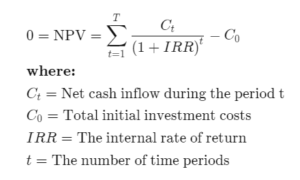### 14) 4 Terms that all Real Estate Investors Should Know### 14) 4 Terms that all Real Estate Investors Should Know

If you’re looking to invest in real estate as a passive investor, you’ll come across a lot of different acronyms. All the jargon can be very confusing, especially for passive investors or newcomers. Here are four terms that are the most important to be aware of when investing in real estate.

CoC (Cash on Cash return)

As I am a big believer of the philosophy that Cash is King, this is an important metric for me. The essence of the CoC is: how much cash will I get back?  Cash-on-Cash return is calculated by dividing the cash you will receive by the cash you have invested. For example, if you invest \$100,000 and receive \$10,000 back, your CoC is calculated as

• CoC=\$10,000/\$100,000=10%

The downside of CoC is what about my principle? At the end of the day if you get \$10,000 back on a \$100,000 investment but don’t get the initial \$100,000 back, you really didn’t get a 10% return, did you? You must keep in mind that the return of your capital has to be secured before you look at a return on your capital.

Cap Rates

Short for capitalization rate. This is a measure of the rate of return that is expected from a property and is calculated as follows:

• Net Operating Income (NOI)/Market Value

The Cap Rate is good to measure compare values of similar properties but doesn’t take into account any loans or expected cash flow from the property. Assume you had two investments that you were looking at. One will give you \$50,000 a year in net operating income and the investment amount is \$900,000. The other will give you \$90,000 a year in net operating income and the investment amount is \$1,100,000. The calculation is as follows:

 Option 1 Option 2 Cost 900,000 1,100,000 Net Operating Income 50,000 90,000 Cap Rate 50,000/900,000 =5.5% 90,000/1,100,000 =8.1%

This method shows one investment produces superior income over another investment and therefore you would choose the higher cap rate. This is of course a very simplified example and there would be a lot of due diligence into the matter, such as how did you arrive at net operating income? What is the potential for growth? And more importantly, what is the cash flow?

ROI/IRR

The ROI is your return on investment. While the CAP rate tells you what your return on investment IS at the current time, the ROI tells you the return on investment over a period of time. CAP rates assume the property is purchased using cash.

ROI assumes the property took a loan and used debt. ROI is calculated by dividing the cash received by the investment amount. Let’s take an example.

• You invest \$100,000 in a property. You put down 10% (10,000) and take a loan for \$90,000. You receive \$7,000 every year in net operating income.
• ROI: \$7,000/\$100,000= 7%
• CoC: \$7,000/\$10,000=70% Cash on cash is higher because the actual cash you invested in \$10,000.

ROI has an evil twin known as IRR (internal rate of return). The reason IRR is an evil twin is because the calculation is much more complicated than ROI. In essence, the IRR identifies the annual growth rate whereas the ROI is total growth from beginning to end.

If you invested \$100,000 and received a \$10,000 return for 5 years calculation would be as follows:

• ROI: 50,000/100,000= 50% over 5 years is 10% per year

However, \$10,000 received this year is not the same value as \$10,000 received in year 5. Therefore, the IRR calculation is used.

The IRR is 8.45% which is less than 10% because of the time value of money.

Here’s the IRR formula, in case you have insomnia one night and you really want to calculate manually. Otherwise, just use an IRR calculator online.EM (Equity Multiple)

The equity multiple is the total cash received divided by the total cash invested, including your initial investment. So, if \$100,000 is invested and you receive \$110,000 back, the calculation is as follows:

• EM=110,000/100,000=1.10X

Basically, this says that you received your initial investment (100%) plus a return of 10%. People tend to confuse this with an ROI or CoC. This does not mean you received a 110% return. Just a 10% return on top of your initial investment. Any number then 1.00x means you are not getting your initial investment back!

Conclusion

These 4 terms are just the introductory into the world of real estate investments. Having an understanding of these key terms will help you become a more educated investor so that you can ask the right questions and understand the full picture for your risks and returns.